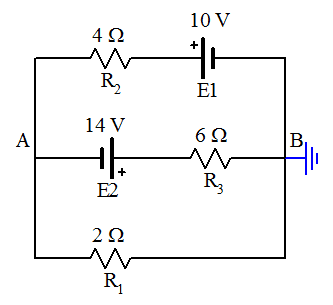# Circuit problem with two voltage source

• terryds

## Homework Statement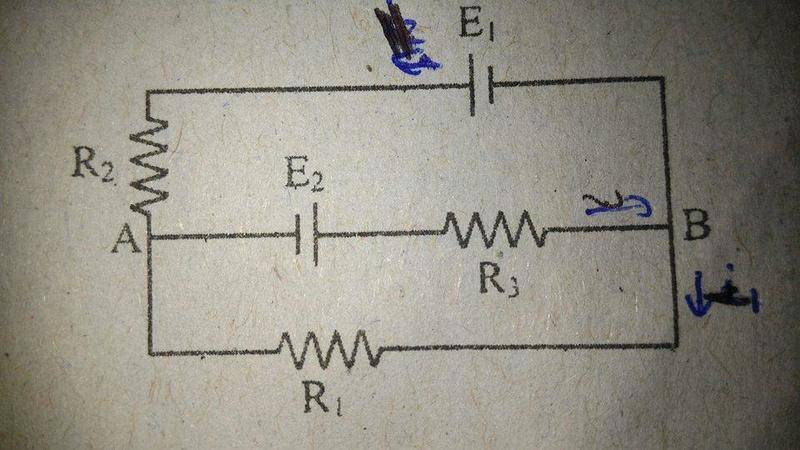R1 = 2 ohms
R2 = 4 ohms
R3 = 6 ohms

E1 = 10 V
E2 = 14 V

What is the voltage between point A and B ?
A. 0.18 V
B. 0.98 V
C. 1.34 V
D. 1.64 V
E. 3.28 V

KVL

## The Attempt at a Solution

[/B]
I tried using KVL
I assume that both mesh currents will go in clock-wise direction.
x = current goes in lower mesh
y = current goes in upper mesh
So, the current that goes through AB or I3 is (x-y)

For the lower mesh,

E2 - I3R3 - x R1
14 - 6(x-y)-2x = 0

For the upper mesh,

4y - 10 - 6 (y-x) - 14 = 0

Solve for x and y..

x = 5.8 A
y = 5.4 A

So, the current goes through AB is 0.4 A

So,
Vb = 14 - 6 (0.4) = 11.6 V
Va = 11.6 - 2(5.8) = 0 V

So, I think Vab = Va - Vb = -11.6 V
But, it's not in the option..

However, this is the book answer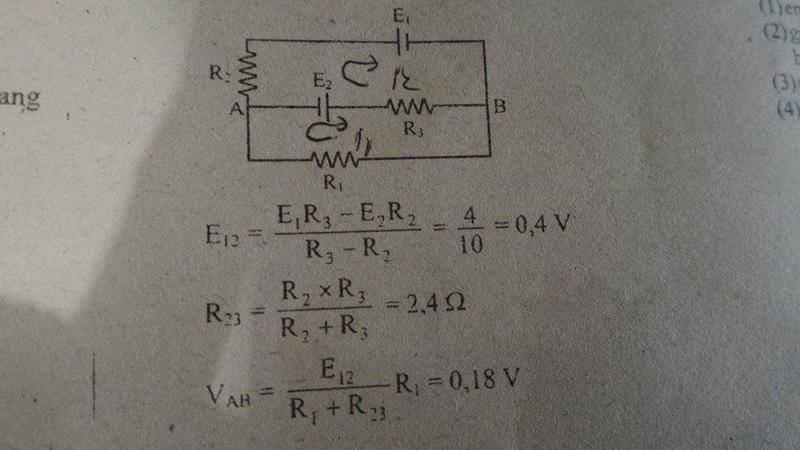I have no idea what methods are used to solve this very quickly.

Last edited:

4y - 10 - 6 (y-x) - 14 = 0
You need to reconsider this equation.

Generally, it is easier if you wait with inserting values until you have the final expression. It makes it much easier to follow what you are doing.

You need to reconsider this equation.

Generally, it is easier if you wait with inserting values until you have the final expression. It makes it much easier to follow what you are doing.

What's wrong with that equation??

Last edited:
What's wrong with that equation??

I'll update my first post
Try doing it again. Mark the direction of the assumed currents at each wire and follow the loops.

Try doing it again. Mark the direction of the assumed currents at each wire and follow the loops.

Alright. I try again.

For the lower mesh,

I start at point A and going clockwise

E2 - I3R3 - I1R1 = 0

E2 - (I1 - I2) R3 - I1R1 = 0

14 - 6 (I1 - I2) - 2 I1 = 0

For the upper mesh,

I also start at point A and going clockwise

- I2R2 - E1 - (I2 - I1)R3 - E2 = 0
- 4 I2 - 10 - 6 (I2 - I1) - 14 = 0

(sorry, I made the mistake at this upper mesh equation)

Solving both equation :

I1 = -0.0909 A
I2 = -2.4545 A

which means that both current goes in counter-clockwise direction, right ? (since I assumed the currents are in clockwise)

I3 = I1-I2 = 2.3636 A

Vab = Vb- Va = I1R11 = 0.0909 A * 2 ohms = 0.1818 V
Thanks for help!

Anyway, what's the name of method my book using? It's so simple and I'd like to learn about it.
Indeed, using calculator is not allowed in the test so I need to know better methods to solve this.

Last edited:
Anyway, what's the name of method my book using? It's so simple and I'd like to learn about it.
Indeed, using calculator is not allowed in the test so I need to know better methods to solve this.
It is Thevenin's theorem. The upper part of the circuit between A and B (with R1 removed) is replaced with a voltage source with emf equal to the open-circuit voltage between A and B and the equivalent resistance RAB, when the sources are replaced with shorts.
http://hyperphysics.phy-astr.gsu.edu/hbase/electric/thevenin.html

•terryds
It is Thevenin's theorem. The upper part of the circuit between A and B (with R1 removed) is replaced with a voltage source with emf equal to the open-circuit voltage between A and B and the equivalent resistance RAB, when the sources are replaced with shorts.
http://hyperphysics.phy-astr.gsu.edu/hbase/electric/thevenin.html

Alright, I'm interested in this approach. I've just watched basics of Thevenin's theorem video.

It says that I should turn the 'mess' into a Thevenin voltage and a Thevenin resistor
In the video, it's asked to determine current, but in my question, it is voltage between A and B, so I'm a bit confused.

So, what is the 'mess' here? I can't decide if it's the upper part or the lower part.

Alright, I'm interested in this approach. I've just watched basics of Thevenin's theorem video.

It says that I should turn the 'mess' into a Thevenin voltage and a Thevenin resistor
In the video, it's asked to determine current, but in my question, it is voltage between A and B, so I'm a bit confused.

So, what is the 'mess' here? I can't decide if it's the upper part or the lower part.
It is the upper part. Ignore R1.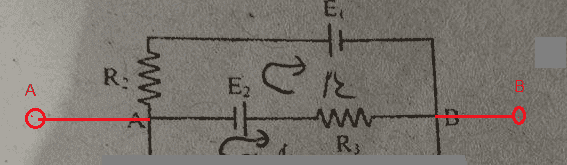Determine the voltage Uo between A and B. That will be the emf of the Thevenin-equivalent voltage source. The internal resistance of the source is obtained if you connect A and B with a zero ohm wire and determine the current Is flowing through that "short". The internal resistance is Ri=Uo/Is. But it is the same as the equivalent resistance between A and B if you replace the batteries by shorts, (simple wires). When you know the emf and internal resistance of the Thevenin-equivalent source, you need connect the resistor R1 to its terminals, and find the voltage across it.

It is the upper part. Ignore R1.

View attachment 101347

Determine the voltage Uo between A and B. That will be the emf of the Thevenin-equivalent voltage source. The internal resistance of the source is obtained if you connect A and B with a zero ohm wire and determine the current Is flowing through that "short". The internal resistance is Ri=Uo/Is. But it is the same as the equivalent resistance between A and B if you replace the batteries by shorts, (simple wires). When you know the emf and internal resistance of the Thevenin-equivalent source, you need connect the resistor R1 to its terminals, and find the voltage across it.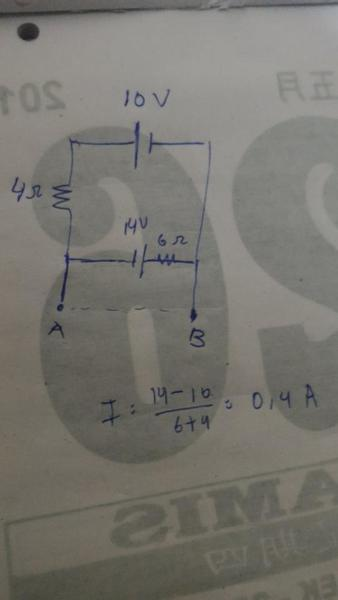Alright, This is what I've done.

I just know how to find the current that loops through the upper part.

EDIT : I made a mistake

The current should be 10+14/(4+6) = 2.4 A

Last edited:
The current should be 10+14/(4+6) = 2.4 A
Yes, it is the current that flows through the resistors, anti-clockwise. Consider the potential zero at A. What is the potential at B?

Yes, it is the current that flows through the resistors, anti-clockwise. Consider the potential zero at A. What is the potential at B?

Since the current goes anti-clockwise, I think there will be potential rise across the 14V E2 and potential drop across 6 ohms resistor.

So, the potential will be = 14 - 2.4 * 6 = -0.4 V, right?

And, for the resistor, I changed all the voltage sources into a short circuit/wire.
Then, I get the resistance is equal to the parallel combination of 6 ohms and 4 ohms, which is 2.4 ohms.
Then, I reconnect the resistor R1 to the Thevenin circuit. So, the voltage across the R1 is 0.4 * 2 / (2 + 2.4) = 0.18 V
Thanks for helping me through this

But, I'm still confused about one thing.
Why the answer is not 0.4 V which is the potential difference between point A and B ?
Why we instead find the potential across R1 resistor ??

And, one more question ._.
Why the book approach in finding the Thevenin emf is just (E1 R3 - E2R2)/(E3-R2)? It seems like a mean(average-value), but why it substracts?
Both E1 and E2 are running currents in the same direction (anti-clockwise), but why it substracts in the book approach? Or, is there a theorem about this?

Last edited:
Since the current goes anti-clockwise, I think there will be potential rise across the 14V E2 and potential drop across 6 ohms resistor.

So, the potential will be = 14 - 2.4 * 6 = -0.4 V, right?

And, for the resistor, I changed all the voltage sources into a short circuit/wire.
Then, I get the resistance is equal to the parallel combination of 6 ohms and 4 ohms, which is 2.4 ohms.
Then, I reconnect the resistor R1 to the Thevenin circuit. So, the voltage across the R1 is 0.4 * 2 / (2 + 2.4) = 0.18 V
Thanks for helping me through this
That looks good!

But, I'm still confused about one thing.
Why the answer is not 0.4 V which is the potential difference between point A and B ?
Why we instead find the potential across R1 resistor ??
Don't forget about the Thevenin resistor in series with the v0.4V source.
And, one more question ._.
Why the book approach in finding the Thevenin emf is just (E1 R3 - E2R2)/(R3 + R2)? It seems like a mean(average-value), but why it substracts?
Both E1 and E2 are running currents in the same direction (anti-clockwise), but why it substracts in the book approach? Or, is there a theorem about this?
Slight typo (corrected in red.)

In relation to points A and B, E1 and E2 are in opposite directions.

•terryds
That looks good!

Don't forget about the Thevenin resistor in series with the v0.4V source.
Slight typo (corrected in red.)

In relation to points A and B, E1 and E2 are in opposite directions.

Can the potential difference between point A and point B be I2R2 + E1 ?? (if we refer to the upper part)

Last edited:
Can the potential difference between point A and point B be I2R2 + E1 ?? (if we refer to the upper part)
Try it & see.

Try it & see.

Yes, it can.. Thanks

•SammyS
Since the current goes anti-clockwise, I think there will be potential rise across the 14V E2 and potential drop across 6 ohms resistor.

So, the potential will be = 14 - 2.4 * 6 = -0.4 V, right?
It is the potential difference between B and A.
And, for the resistor, I changed all the voltage sources into a short circuit/wire.
Then, I get the resistance is equal to the parallel combination of 6 ohms and 4 ohms, which is 2.4 ohms.
Then, I reconnect the resistor R1 to the Thevenin circuit. So, the voltage across the R1 is 0.4 * 2 / (2 + 2.4) = 0.18 V
Thanks for helping me through this

But, I'm still confused about one thing.
Why the answer is not 0.4 V which is the potential difference between point A and B ?
Why we instead find the potential across R1 resistor ??
0.4 V was the voltage without load, the emf of the Thevenin source. If you connect the resistor R1, the current flowing will cause potential drop across the internal resistor and the voltage on R1 becomes less than the emf. It happens every time you load a voltage source. The terminal voltage is always less than the emf.
And, one more question ._.
Why the book approach in finding the Thevenin emf is just (E1 R3 - E2R2)/(E3-R2)? It seems like a mean(average-value), but why it substracts?
Both E1 and E2 are running currents in the same direction (anti-clockwise), but why it substracts in the book approach? Or, is there a theorem about this?
You would get the same formula if you did the derivation symbolically. I=(E1+E2)/(R3+R2) , UAB=E1-R2I or UAB=R3I-E2. Bring to common denominator and simplify.

•terryds
I have no idea what methods are used to solve this very quickly.

Have you considered applying nodal analysis (KCL) rather than loop analysis?

A very quick solution can be obtained if you apply nodal analysis. If you choose the reference node to be B then A is the only essential node. That means you can solve for the potential at A with respect to B using a single node equation.

Have you considered applying nodal analysis (KCL) rather than loop analysis?

A very quick solution can be obtained if you apply nodal analysis. If you choose the reference node to be B then A is the only essential node. That means you can solve for the potential at A with respect to B using a single node equation.

##\frac{V_b-E_1}{R_2} = \frac{V_b-E_2}{R_3+R_1} \\
\frac{(V_b-10)}{4} = \frac{(V_b-14)}{8}##

But, I get ##V_b## equals to 6 V.

##\frac{V_b-E_1}{R_2} = \frac{V_b-E_2}{R_3+R_1} \\
\frac{(V_b-10)}{4} = \frac{(V_b-14)}{8}##

But, I get ##V_b## equals to 6 V.